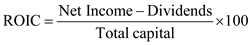# Quiz 21: Mavis Machine Shop

Calculate the pretax ROIC: Pretax ROIC: Return on invested capital is the return that any company, firm, or an individual earns on the amount of capital invested over a period of time. The pretax return on invested capital (ROIC) can be calculated by using the following formula:For wholesaler channel estimate: Substitute: For net income as \$4, dividends as \$0 and total capital as \$13Thus, the return on invested capital is 30.76%. For original Levi's store channel estimate: Substitute: For net income as \$6, dividends as \$0, and total capital as \$38Thus, the return on invested capital is 15.78%.